# Aggregate Daily Data to Month & Year Intervals in R (2 Examples)

In this R tutorial you’ll learn how to summarize and group daily data into monthly intervals.

The tutorial will contain two examples for the aggregation of daily data. More precisely, the article will consist of this content:

Let’s just jump right in…

## Introducing Example Data

Consider the following example data:

```set.seed(8965379) # Create random example data data <- data.frame(date = sample(seq(as.Date("2020/01/01"), by = "day", length.out = 1000), 100, replace = TRUE), value = round(rnorm(100, 5, 2), 2)) head(data) # Print head of example data```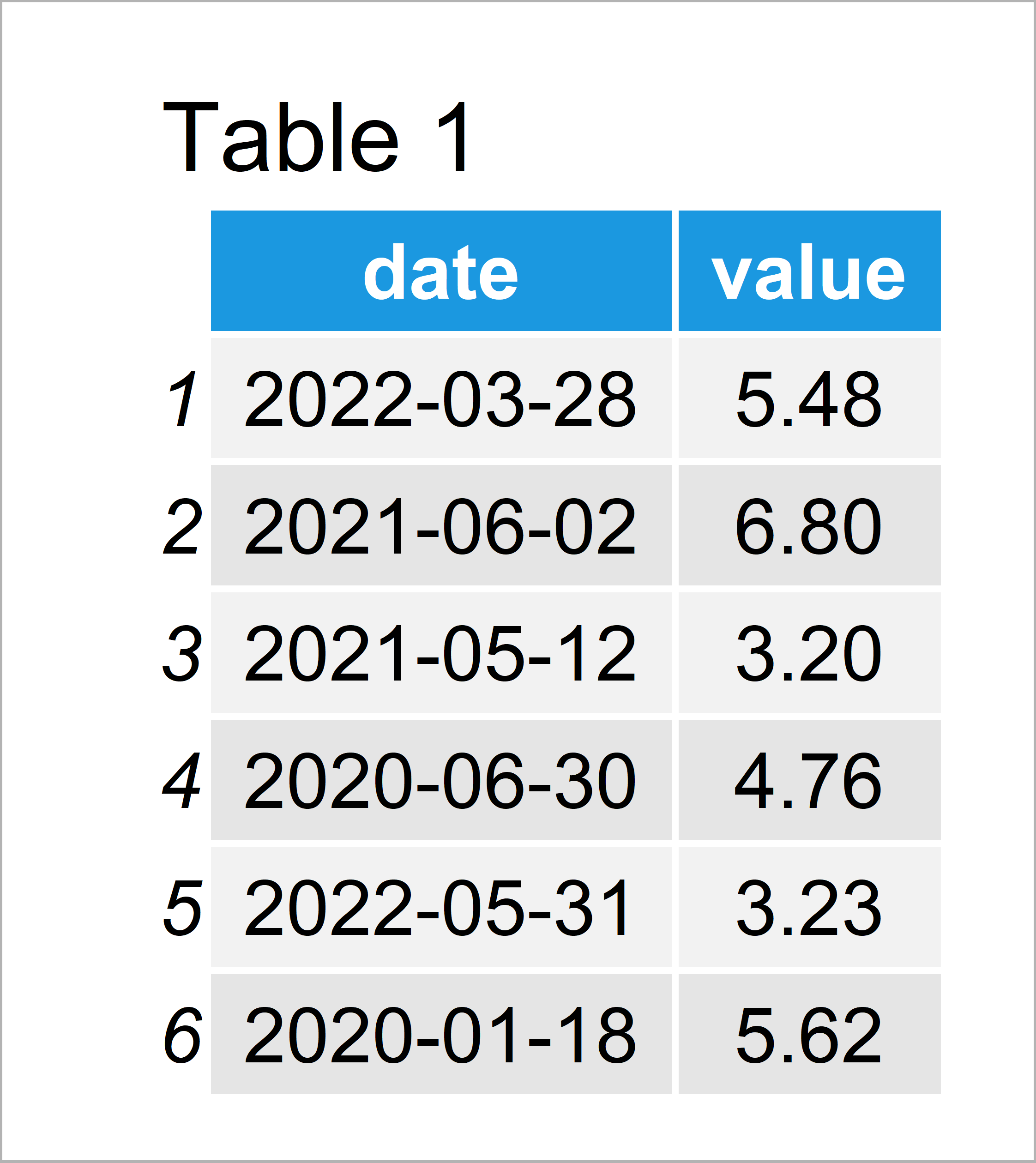Table 1 shows the structure of our example data: It is composed of 100 data points and two columns. The first variable contains a random sequence of dates and the second variable contains corresponding values.

## Example 1: Aggregate Daily Data to Month/Year Intervals Using Base R

The following R syntax explains how to use the basic installation of the R programming language to combine our daily data to monthly data.

First, we have to add a year and a month column to our data frame:

```data_new1 <- data # Duplicate data data_new1\$year <- strftime(data_new1\$date, "%Y") # Create year column data_new1\$month <- strftime(data_new1\$date, "%m") # Create month column head(data_new1) # Head of updated data```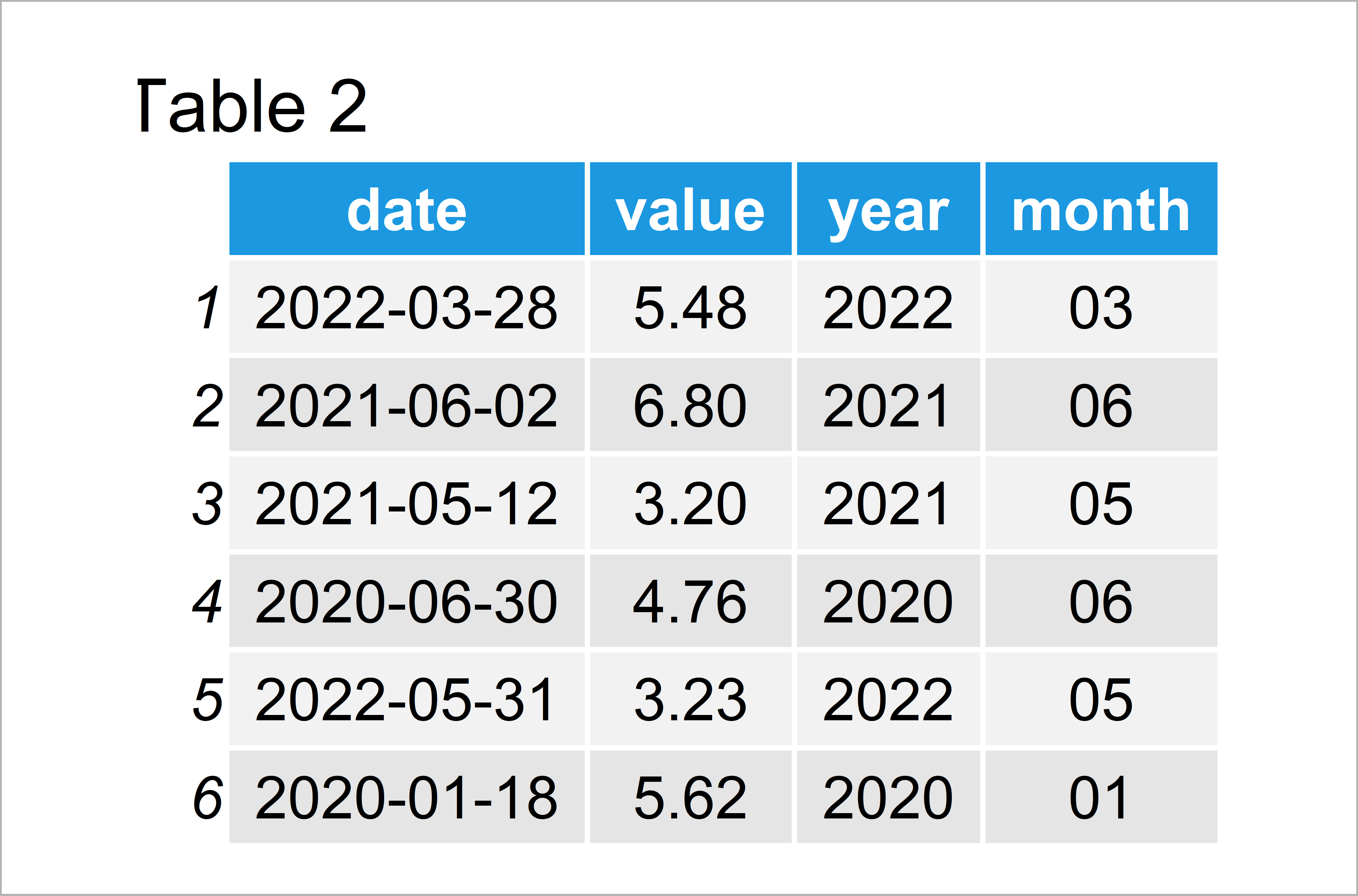Table 2 shows the output of the previous R programming syntax: We have created a new data frame containing separate year and month columns.

In the next step, we can apply the aggregate function to convert our daily data to monthly data:

```data_aggr1 <- aggregate(value ~ month + year, # Aggregate data data_new1, FUN = sum) head(data_aggr1) # Head of aggregated data```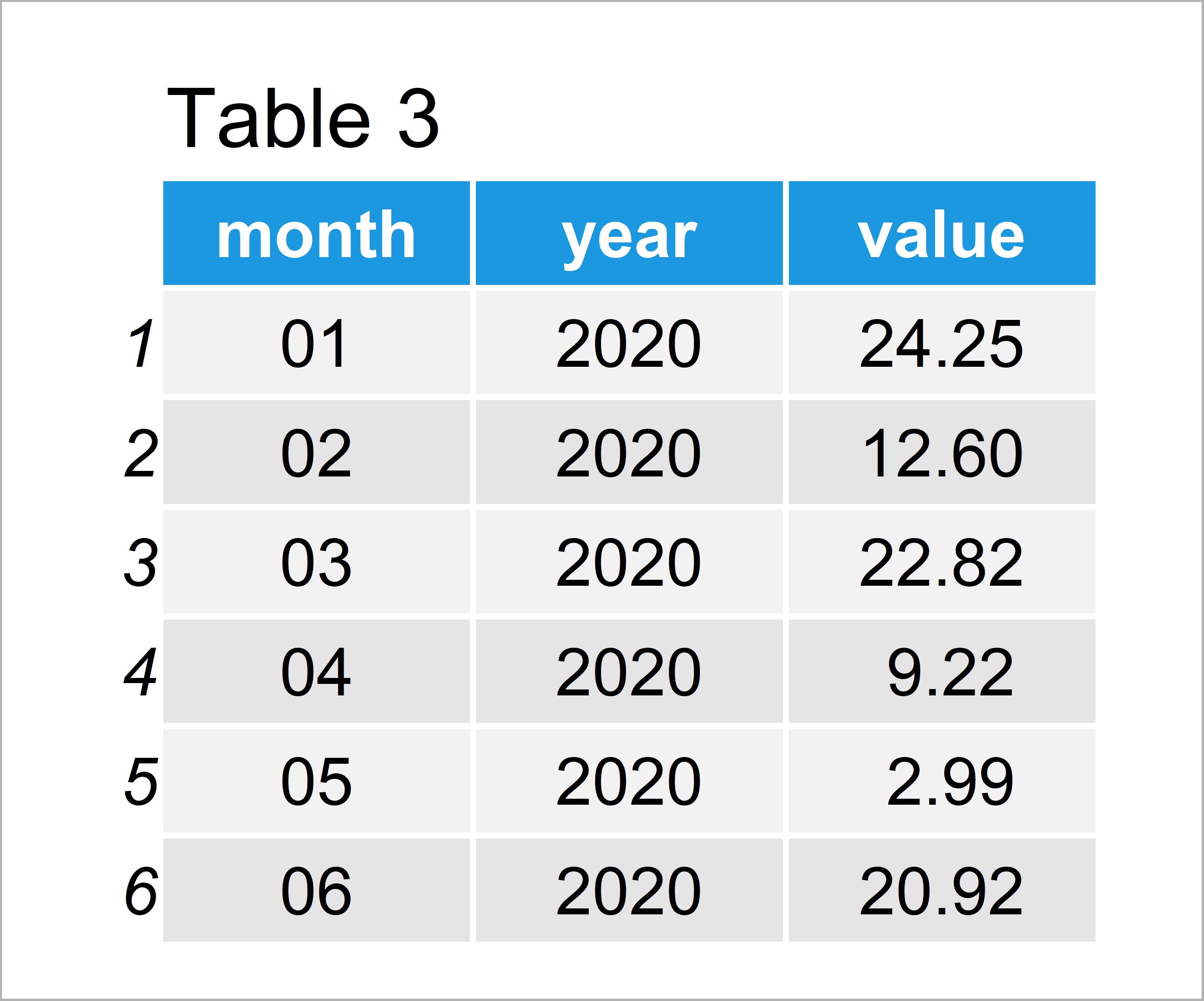Table 3 illustrates the output of the previous R syntax: An aggregated version of our data frame.

In this case, we have used the sum function to get the sum of all values within each month. However, you may also use other functions such as mean or median to summarize our data.

## Example 2: Aggregate Daily Data to Month/Year Intervals Using lubridate & dplyr Packages

Example 2 illustrates how to use the functions of the tidyverse environment to switch from daily to monthly/yearly data.

First, we need to install and load the lubridate package:

```install.packages("lubridate") # Install & load lubridate library("lubridate")```

Now, we can use the floor_date function to add a year/month column to our data frame:

```data_new2 <- data # Duplicate data data_new2\$year_month <- floor_date(data_new2\$date, # Create year-month column "month") head(data_new2) # Head of updated data```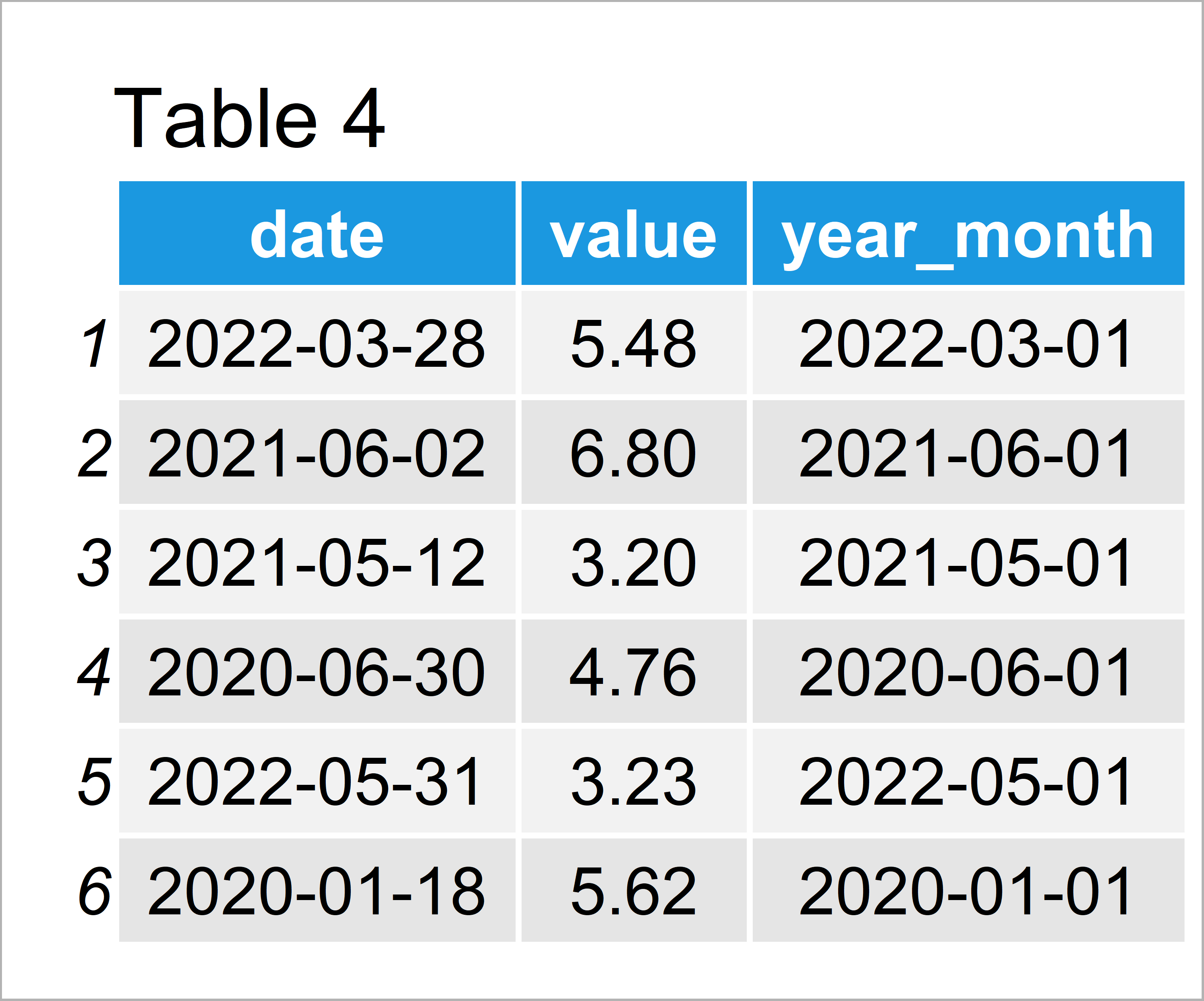As revealed in Table 4, the previous R code has managed to construct a new data frame containing a year/month variable. Note that our year/month variable still contains days, but all days were set to 01.

Next, we have to install and load the dplyr package to R:

```install.packages("dplyr") # Install dplyr package library("dplyr") # Load dplyr```

Now, we can use the group_by and summarize functions of the dplyr package to aggregate our data.

```data_aggr2 <- data_new2 %>% # Aggregate data group_by(year_month) %>% dplyr::summarize(value = sum(value)) %>% as.data.frame() head(data_aggr2) # Head of aggregated data```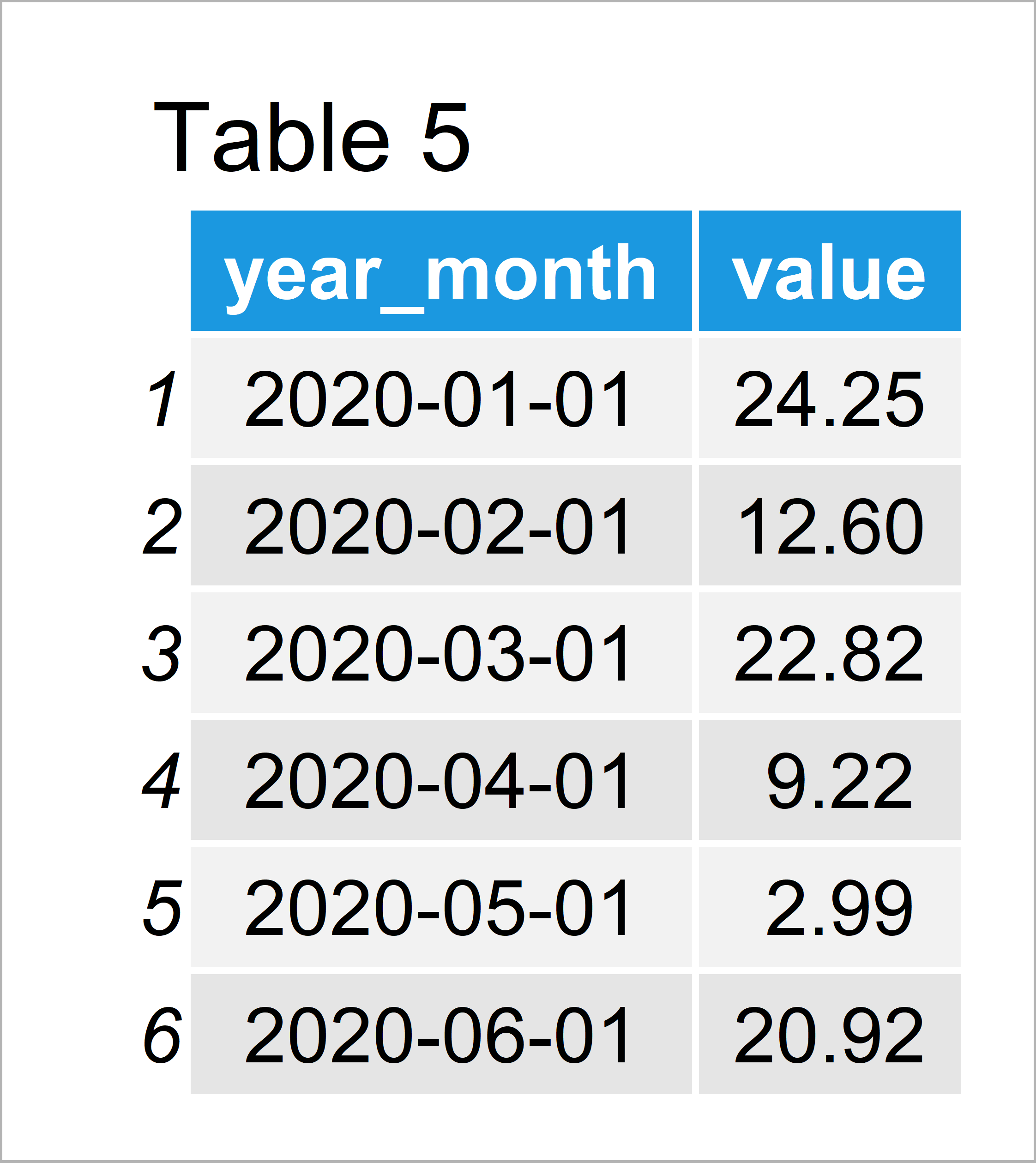In Table 5 it is shown that we have created an aggregated data frame by running the previous R programming syntax.

The data shown in Table 5 is exactly the same as in Table 3. Whether you want to use the functions of Base R or the tidyverse environment is a matter of taste.

## Video, Further Resources & Summary

Some time ago I have published a video on my YouTube channel, which shows the R syntax of this tutorial. Please find the video below:

Please accept YouTube cookies to play this video. By accepting you will be accessing content from YouTube, a service provided by an external third party.If you accept this notice, your choice will be saved and the page will refresh.

In addition, you might have a look at some of the related articles of this website.

You have learned in this tutorial how to aggregate time series data from daily to monthly/yearly in the R programming language. If you have additional questions, let me know in the comments below. Furthermore, please subscribe to my email newsletter in order to receive regular updates on new tutorials.

Subscribe to the Statistics Globe Newsletter